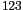DATA MINING
Desktop Survival Guide
by Graham Williams# Samples

Rattle uses a simple approach to generating a partitioning of our dataset into training and testing datasets with the sample function.

 ```crs\$sample <- sample(nrow(crs\$dataset),floor(nrow(crs\$dataset)*0.7)) ```

The first argument to sample is the top of the range of integers you wish to choose from, and the second is the number to choose. In this example, corresponding to the audit dataset, 1400 (which is 70% of the 2000 entities in the whole dataset) random numbers between 1 and 2000 will be generated. This list of random numbers is saved in the corresponding Rattle variable, `crs\$sample` and used throughout Rattle for selecting or excluding these entities, depending on the task.

To use the chosen 1400 entities as a training dataset, we index our dataset with the corresponding Rattle variable:

 ```crs\$dataset[crs\$sample,] ```

This then selects the 1400 rows from `crs\$dataset` and all columns.

Similarly, to use the other 600 entities as a testing dataset, we index our dataset using the same Rattle variable, but in the negative!

 ```crs\$dataset[-crs\$sample,] ```

Each call to the sample function generates a different random selection. In Rattle, to ensure we get repeatable results, a specific seed is used each time, so that with the same seed, we obtain the same random selection, whilst also providing us with the opportunity to obtain different random selections. The set.seed function is called immediately prior to the sample call to specify the user chosen seed. The default seed used in Rattle is arbitrarily the number:

 ```set.seed(123) crs\$sample <- sample(nrow(crs\$dataset),floor(nrow(crs\$dataset)*0.7)) ```

In moving into R we might find the sample.split function of the caTools package handy. It will split a

vector into two subsets, two thirds in one and one third in the other, maintaining the relative ratio of the different categoric values represented in the vector. Rather than returning a list of indices, it works with a more efficient Boolean representation:

 ```> library(caTools) > mask <- sample.split(crs\$dataset\$Adjusted) > head(mask)  TRUE TRUE TRUE FALSE TRUE TRUE > table(crs\$dataset\$Adjusted) 0 1 1537 463 > table(crs\$dataset\$Adjusted[mask]) 0 1 1025 309 > table(crs\$dataset\$Adjusted[!mask]) 0 1 512 154 ```

Perhaps it will be more convincing to list the proportions in each of the groups of the target variable (rounding these to just two digits):

 ```> options(digits=2) > table(crs\$dataset\$Adjusted)/ length(crs\$dataset\$Adjusted) 0 1 0.77 0.23 > table(crs\$dataset\$Adjusted[mask])/ length(crs\$dataset\$Adjusted[mask]) 0 1 0.77 0.23 > table(crs\$dataset\$Adjusted[!mask])/ length(crs\$dataset\$Adjusted[!mask]) 0 1 0.77 0.23 ```

Roption[digits] Thus, using this approach, both the training and the testing datasets will have the same distribution of the target variable.